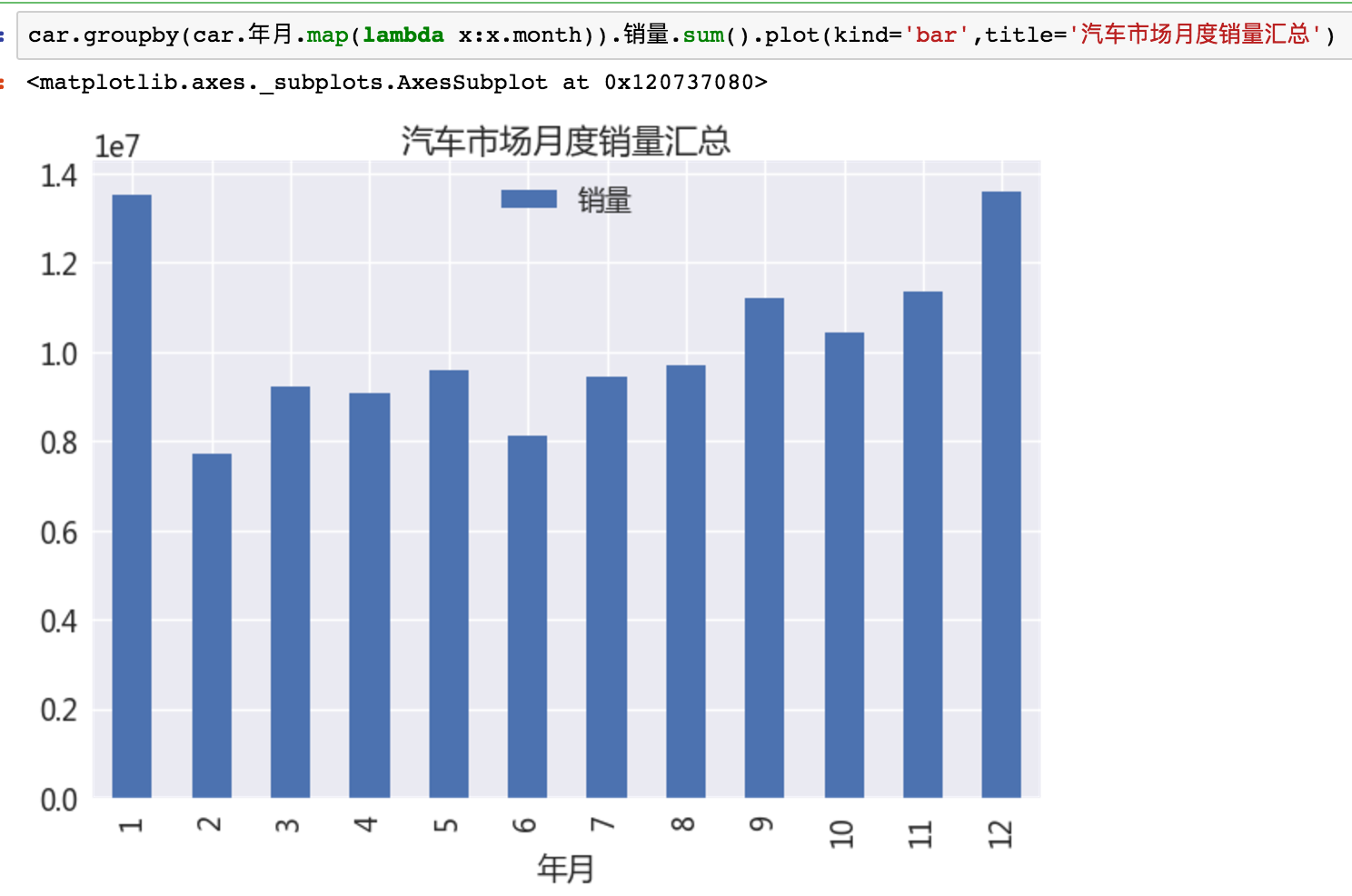### 用python怎么做数据统计-Python数据分析之路（一）查询和统计

0. 如何入门数据分析

1. 找到好的问题2. 查询和过滤

DataFrame是pandas的核心数据结构，可以理解为Excel里的二维矩阵，它更高级，能表达3维或更高维的数据，支持多索引。在内存中存取，效率极高，绝大多数操作都和DataFrame相关。维度为2的DataFrame，行(column)和列(row)的axis分别为0和1。可以针对某些列做索引(index),高维DataFrame是相当少见的。

2.1 查询

[]

df[‘房价’] ,df[[‘房价’,’车价’]]

loc

df.loc, df[‘2013′:’2015’]

iloc

df.iloc, df.iloc[2:10]

ix

df.ix, df.ix[2:10]

weather.index= weather[u’日期’].map(lambda x:Timestamp(x))

Pandas的索引功能非常强大，补充如下：

loc也能支持先行后列的查询：df.loc[‘20130101′:’20130103’ , [‘A’,’B’]],类似的如iloc

2.2 过滤

df=df[!data.col.isnull()]

db[db.city.str.contains(u’市’)]

qq[‘id’].unique()

isin能判断单元格中的值是否在给定的数组内，若希望对多个列做过滤，Pandas提供了现成的方法df.filter，还支持正则。还能进行逻辑操作，实现更复杂的需求。

2.3 遍历

lambda参数

map

cell

apply

axis:不填写cell,1:行,2:列

applymap

cell

cell

element-wise最为灵活

iterrows

iteritems

itertuples

map, apply,applymap是只返回单元格或行列本身的，参数都是lambda,本节假设读者对python的lambda表达式有足够的了解。

for i,row in data.iterrows():

pass

2.4 求值和合并

table[‘面积’]= np.round(table[‘总价’]*10000/table[‘单价’])

numpy提供了绝大多数常见的函数算子，能满足大部分需求。下面是合并：

data.merge(right=prop_rates, how=’inner’,left_on=’Property_Area’,right_index=True, sort=False)

3. 分组，排序，聚合

3.1 分组

df2.groupby(df2.日期.map(lambda x:x.year))

Pandas也能支持传递多个列的数组，除了切片以外，能在索引上使用的基本都能在group,sort上使用，一致性的API上手非常容易。

db[db.city==u’北京’][u’平均值’].resample(’12M’).aqi.mean()

3.2 排序

Pandas的排序非常之快，大部分操作都能在瞬间完成。排序分为两类：

groupby之后的数据，和一般的DataFrame不同，而像个字典(dict)。对键排序，需使用sort_index，值排序，需使用sort_values。

3.3 聚合

df2.groupby(df2.日期.map(lambda x:x.year)).size()

car.groupby(car.年月.map(lambda x:x.month)).销量.sum().plot(kind=’bar’,title=’汽车市场月度销量汇总’)Pandas支持直接将聚合结果绘图输出（虽然丑但是方便啊），下一节我们将详细介绍它的使用细节。

df[(df.省==’广西’) & (df.车型分类==’MPV’) & (df.品牌==’东风’)].groupby(‘车型’).size().sort_values(ascending=False)

3.4 数据透视表

pd.pivot_table(df,index=[“Name”,”Rep”,”Manager”],values=[“Price”],aggfunc=[np.sum,len],fill_value=0)pivot之后，生成的DataFrame是multiindex的，处理起来稍显繁琐，用xs可将某个子index的数据”提升”出来，例如:

df_pivot.xs((’12AM新坐标’,2011))

4. 总结

Pandas本身异常强大，功能非常繁杂，笔者仅仅掌握了其中非常小的一部分。但是对于一般的需求都能通过简单的操作组合出来。API的一致性非常重要，Pandas（包括numpy等）都继承了Python的优良特性，因此只要能举一反三，就能进步神速。